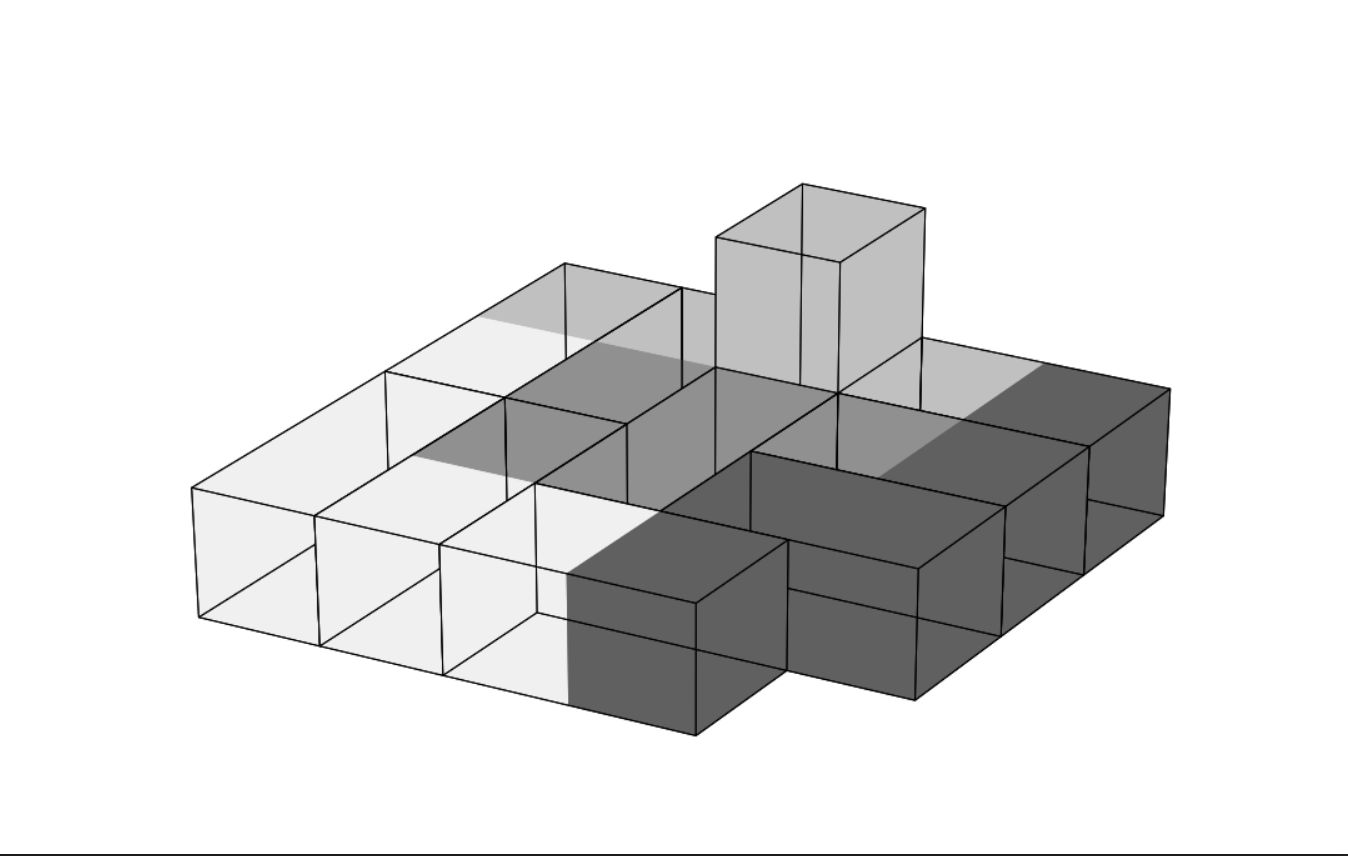시간 제한메모리 제한제출정답맞힌 사람정답 비율
1 초 (추가 시간 없음) 256 MB42250.000%

## 문제

Mr. Panda recently received a bucket of toy blocks as his birthday gift. Each block is a $1 \times 1\times 2$ cuboid, which is constructed by a pair of face-to-face $1 \times 1 \times 1$ colored cubes. There are $n$ types of colors, labeled as $1, 2, \ldots, n$.

Mr. Panda checked all of the blocks, and he found that he had just $\frac{n \times (n+1)}{2}$ blocks and each of these blocks was painted with a unique pair of colors. That is, for each pair of colors $(i, j)$ $(1 \leq i \leq j \leq n)$, he had exactly one block with one cube colored $i$, and the other colored $j$.

Mr. Panda plans to build a fantastic castle with these blocks today.Firstly, he defines an attribute called connected:

1.  If cube A shares a face with cube B, they are connected.
2.  If cube A and cube B are connected, and cube B and cube C are connected, then cube A and cube C are connected.
3.  If all pairs of cubes in the castle are connected, the castle is connected.

Then he comes up with the following requirements:

1. The whole castle should be connected.
2. For any color $i$, if only consider the cubes with that color, the sub-castle formed by these cubes should also be connected.

However, after many attempts, Mr. Panda still cannot build such a castle. So he turns to you for help. Could you please help Mr. Panda to build a castle which meets all his requirements?

## 입력

The first line of the input gives the number of test cases, $T$ ($1 \leq T \leq 10$). $T$ test cases follow.

For each test case, one line contains an integer $n$ ($1 \leq n \leq 200$), representing the number of colors.

## 출력

For each test case, first output one line containing "Case #x:", where x is the test case number (starting from 1).

If it's impossible to build a castle that satisfies Mr. Panda's requirements, output a single line containing "NO" (quotes for clarity).

If it's possible to build the castle, first output a single line containing "YES" (quotes for clarity).

Then, output $\frac{n \times (n+1)}{2}$ lines describing the coordinates of all the blocks. Each of these lines should be outputted in the form of $i,j,x_i,y_i,z_i,x_j,y_j,z_j$ $(1 \leq i \leq j \leq n, 0 \leq x_i, y_i, z_i, x_j, y_j, z_j \leq 10^{9}$), which means for the block $(i,j)$, the cube with color $i$ is located at $(x_i,y_i,z_i)$ and the other cube with color $j$ is located at $(x_j,y_j,z_j)$. You should make sure that each pair of $(i, j)$ occurs exactly once in your answer.

In case there is more than one solution, any of them will be accepted.

## 예제 입력 1

2
3
4


## 예제 출력 1

Case #1:
YES
1 1 1 1 0 1 2 0
1 2 1 3 0 1 4 0
1 3 2 1 0 3 1 0
2 2 2 2 0 2 3 0
2 3 2 4 0 3 4 0
3 3 3 2 0 3 3 0
Case #2:
YES
1 2 1 3 0 1 4 0
1 1 1 2 0 1 1 0
1 3 2 1 0 2 2 0
2 3 2 4 0 2 3 0
1 4 3 1 0 4 1 0
3 3 3 2 0 3 3 0
2 2 3 4 0 3 4 1
4 4 4 2 0 5 2 0
3 4 4 3 0 5 3 0
2 4 4 4 0 5 4 0